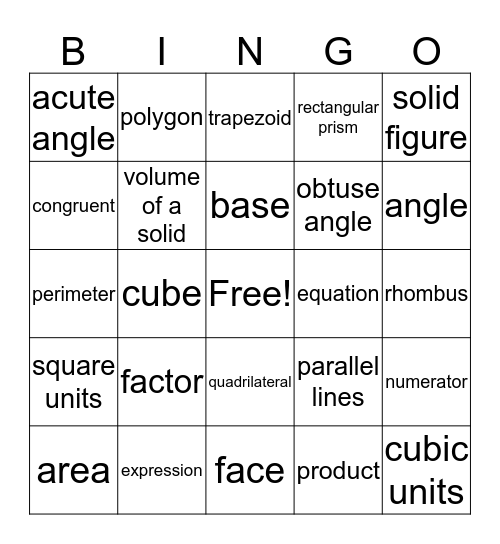# Math Vocabulary BingoThis bingo card has a free space and 24 words: factor, expression, base, area, solid figure, acute angle, congruent, polygon, equation, quadrilateral, obtuse angle, perimeter, parallel lines, numerator, volume of a solid, product, rhombus, trapezoid, angle, cube, cubic units, square units, rectangular prism and face.

## Play Online